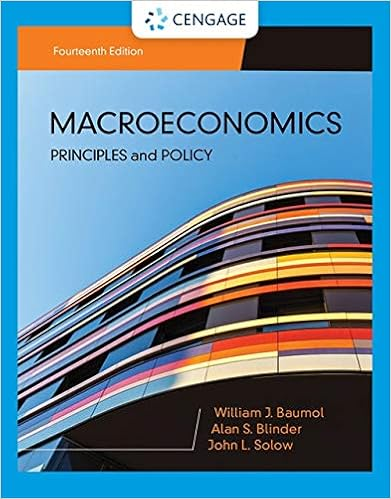# Homework 6 - Maximum number of choices allowed is{0...

• Homework Help
• 21
• 97% (125) 121 out of 125 people found this document helpful

This preview shows page 1 - 4 out of 21 pages.

##### We have textbook solutions for you!
The document you are viewing contains questions related to this textbook.The document you are viewing contains questions related to this textbook.
Chapter 9 / Exercise 2
Macroeconomics: Principles & Policy
Baumol/BlinderExpert Verified
Maximum number of choices allowed is {0}.Submitted by ZEHLER, IAN (IFZ5002) on 4/15/2015 10:21:16 PMSelect regrade mode Choose a regrade mode Change points or correct answer Drop question Give full credit This regrade will affect all users who submitted this Assessment containing this question. Regrade not available This is a linked Assessment or Question. Only the owner has edit rights. Regrade not available This content item's settings prohibit regrade on this item. Points Awarded74.50Points Missed25.50Percentage74.5%1. Consider the following modeli) C = 1500 + mpc (Y - tY)ii) I = 800iii) G = 500Ok CancelCancelCancel
##### We have textbook solutions for you!
The document you are viewing contains questions related to this textbook.The document you are viewing contains questions related to this textbook.
Chapter 9 / Exercise 2
Macroeconomics: Principles & Policy
Baumol/BlinderExpert Verified
iv) X - M = 500 - mpi (Y)where:t = the (flat) tax ratempc = the marginal propensity to consumempi = the marginal propensity to importsuppose mpc = .80, t = .25, mpi = .2Given the information above, solve for the equilibrium output:
Table for Individual Question FeedbackPoints Earned: 3.0/3.0
2. We know that the formula for the (government) spending multiplier is 1/(1-mpc(1-t) + mpi). The value of the government spending multiplier in this problem is: Round to 2 decimal places.
Table for Individual Question FeedbackPoints Earned: 3.0/3.0
3. When we discussed the multiplier we discussed the impact effect. For example, suppose that G increases by 100 to 600 and we assume, as we often do, that firms match the increase in demand by increasing Y by 100. In round two, this is an increase in income of 100 to consumers. We will trace out exactly where this 100 increase in income goes in the second round. Recall, there are three leakages to address (via taxes, imports and savings).Given that t=.25, we know that .25 of every dollar increase in gross income is a leakage due to taxes. Since the increase in income is \$100, we know the leakage due to taxes is:
100 we have the following: Y up by 100, savings up by 20, taxes up by 25, imports up by 20, consumption up by 35Table for Individual Question FeedbackPoints Earned: 3.0/3.0
•••